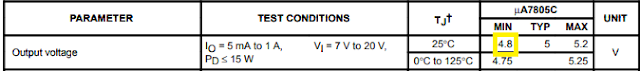top of page

# Calculate Power Dissipation And Junction Temperature In a Linear Voltage Regulator

Updated: Jul 19, 2020

## INTRODUCTION

A linear regulator is a system used to maintain a steady voltage. The resistance of the regulator varies in accordance with the load resulting in a constant output voltage. The regulating device is made to act like a variable resistor, continuously adjusting a voltage divider network to maintain a constant output voltage and continually dissipating the difference between the input and regulated voltages as waste heat. Linear regulators are simple voltage regulator circuits commonly used in electronics. In this article, we will study how to calculate power or heat dissipation taking place in a linear regulator.

## Linear Regulator Internal structure

To understand how to calculate power dissipation taking place in a linear regulator let us first understand the internal structure of a linear regulator. The figure below shows a typical circuit fabricated in a linear regulator IC.Typical Circuit in a Linear Voltage Regulator

As we can see the Linear regulator has Series pass transistor Q1 between its Input Pin and Output Pin. The collector is connected to the unregulated input and the emitter is connected to the load resistor. So the voltage drop across the Q1 is Input voltage minus the output voltage i.e (Vin - Vout).

The current flowing from collector to emitter i.e the collector current of the transistor is the same as the load current Iload. Thus the power dissipation taking place in the pass transistor is:

P = (Vin - Vout)* Iload

This equation gives us the power dissipation in the Linear voltage regulator.

## Example

For linear regulator LM7805, the Input voltage for the application is in range of 12V to 16V, and the Load current is 50mA. Calculate the power dissipation and maximum junction temperature when the ambient temperature is 25°C. Consider TO-220 Package.

Solution:

For 7805 IC the minimum expected output voltage at 25°C is 4.8V as per datasheet. This can be seen in the below image. The given load current is 50mALM7805 datasheet value for minimum output voltage

So the maximum power dissipation taking place in the IC according to the above formula is:

P = (16 - 4.8) *50mA = 560mW

Now to calculate the maximum junction temperature we need to get the thermal resistance (junction to ambient) of the TO-220 package from the datasheet. This can be seen from the image below:Thermal resistance of TO-220 Package

Thus we have thermal resistance Rtja = 19 °C/W and ambient temperature is 25°C.

The Junction temperature can be calculated as: Tj = Ta + Rtja *P

Solving we get Tj = 25 + 19* 0.56 = 35.64 °C

See All
bottom of page